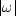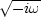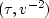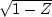Next: GEOMETRY-BASED DECON Up: PIXEL-PRECISE VELOCITY SCANNING Previous: Smoothing in velocity

## Rho filter

Notice the dark halo around the reconstruction in Figure 3. It was suppressed in Figure 5 by the subroutine halfdifa(). Recall that slant-stack inversion (see IEI for an example) requires anfilter. Without doing any formal analysis I guessed that the same filter would be helpful here because the dark halo has a strong spectral component atwhich would be extinguished by anfilter. Thefilter is sometimes called a rho filter.''

Because of the close relation of slant-stack inversion to wave propagation and causality, I found it appealing to factorinto a causalpart and an anticausalpart. I applied a causalafter generating the (t,x)-space and an anticausalbefore making the-space. I implemented the causality by taking the square root of a Fourier domain representation of causal differentiation, namely,.I show this in subroutine halfdifa().

# Half order causal derivative.  OK to equiv(xx,yy)
#
real omega,                       xx(n), yy(n)
complex cz, cv(4096)
n2=1; while(n2<n) n2=2*n2;         if( n2 > 4096) call erexit('halfdif memory')
do i= 1, n2 {             cv(i) = 0.}
do i= 1, n
if( adj == 0)   { cv(i) = xx(i)}
else            { cv(i) = yy(i)}
call ftu( +1., n2, cv)
do i= 1, n2 {
omega = (i-1.) * 2.*3.14159265 / n2
cz = csqrt( 1. - cexp( cmplx( 0., omega)))
if( adj != 0)   cz = conjg( cz)
cv(i) = cv(i) * cz
}
call ftu( -1., n2, cv)
do i= 1, n
if( adj == 0)   { yy(i) = yy(i) + cv(i)}
else            { xx(i) = xx(i) + cv(i)}
return; end


Notice also that vspray() includes a scaling variable named scale. I have not developed a theory for this scale factor, but if you omit it, amplitudes in the reconstructions will be far out of amplitude balance with the input.Next: GEOMETRY-BASED DECON Up: PIXEL-PRECISE VELOCITY SCANNING Previous: Smoothing in velocity
Stanford Exploration Project
10/21/1998